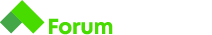# Not Divisible Number

Здравейте, бихте ли ми помогнали с този код, влизам в infinite loop пък и не получавам верен отговор?

Условието:
Write a program that reads from the console a positive integer N and prints all the numbers from 1 to N not divisible by 3 or 7 , on a single line, separated by a space.

## Input

• Will always consists of one valid integer number - the number N .

## Output

• Should always consists of the numbers from 1 to N , which are not divisible by 3 or 7 , separated by a whitespace.

``````  let n = +gets();
let m = "";
let i = 1;
while (i < n){
if (i % 3 === 0 || i % 7 === 0) {
continue;
}
i++;
m += i + " ";
}

print(m, n);``````

Здравей, Васко!

В случаите, когато знаем точно колко итерации, трябва да направим (от 1 до N) е по удачно да използваме for цикъл. По този начин, ще си спестиш декларирането на още една допълнителна променлива i, която е част от сигнатурата на самия for цикъл, а и няма да мислиш къде да инкрементираш i. В твоето решение, първоначално i = 1 и понеже е по-малко от N влизаш в тялото на цикъла, след което проверяваш дали i % 3 === 0 || i % 7 === 0, и понеже и двете проверки връщат false, не влизаш в тялото на проверката, инкрементираш i с едно и присвояваш стойност на m = '2 ', а би трябвало и 1 да запазим като неделимо на 3 и 7 число.
Когато i вече има стойност 3, цикъла ти става безкраен понеже на всяка следваща итерация влизаш в проверката и продължава изпълнението на програмата, просто i вече не се инкрементира никога.

Поздрави,
Иван!

Благодаря ви, ето го и с for loop и пак не ми се получи:

``````let n = +gets();
let m = "";
for (let i = 1; i < n; i++){
if (i % 3 === 0 || i % 7 === 0) {
continue;
}
m += i + " ";
}
print(m,n);``````

Мисля, че ако итерираш до i <= n и ако принтираш само m, без n, решението ти е правилно!

Поздрави,
Иван!

Да получи се благодаря ви!